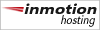#### Pythagoran vakio √2

Jonka muistamme lähes ulkoa.

 √2 = 1.41421356237309504880168872420969807856967187537694... The diagonal of a square of unit side. Pythagoras' Constant.1)

He is unworthy of the name of man who is ignorant of the fact that the diagonal of a square is incommensurable with its side.  Plato (427-347 BC)

There are certainly people who regard √ 2 as something perfectly obvious but jib at √ -1. This is because they think they can visualise the former as something in physical space but not the latter. Actually √ -1 is a much simpler concept.
Edward Charles Titchmarsh (1899-1963).

P. Sebah also gave tiny codes for the computations of √ 2
and produces the following program :

```                    main(){
int a=1000,b=0,c=1413,d,f,n=800,k;
for(;b<c;f[b++]=14);
for(;n--;d+=*f*a, printf("%.3d",d/a),*f=d%a)
for(d=0,k=c;--k;d/=b,d*=2*k-1)
f[k]=(d+=f[k]*a)%(b=100*k);
}
```
1) Wolfram Mathworld

Powered Byand CodeIgniter 3.1.11